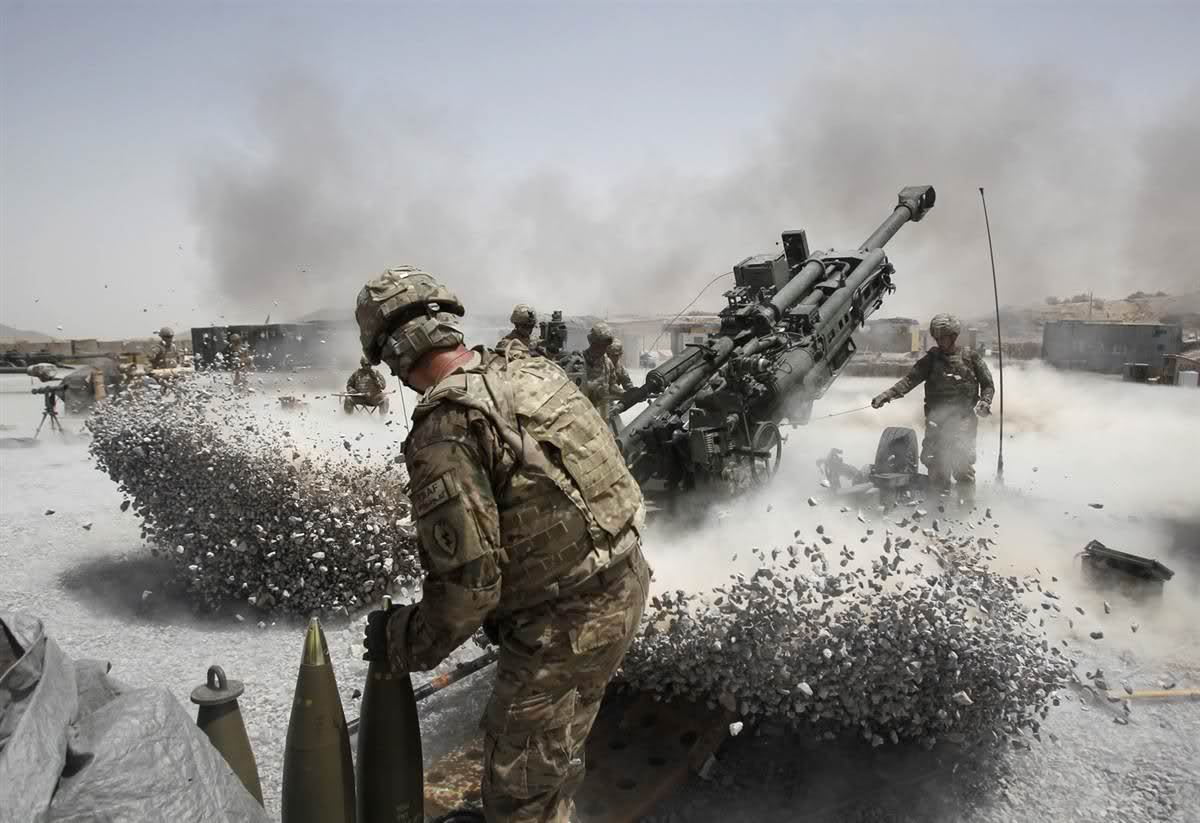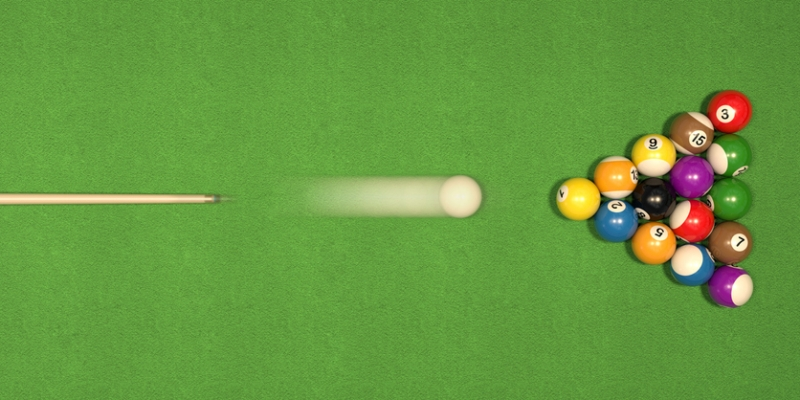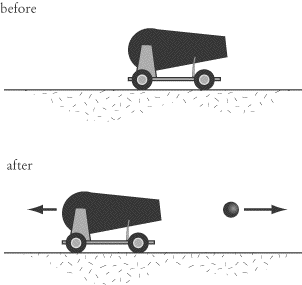Crystal

MS-PS2-2

# Conservation Of Momentum In One Dimension Study Guide

In this chapter, we will study the law of conservation of momentum and how it governs colliding and exploding systems.

Table of content

# IntroductionSource

If you have seen videos of artillery guns being fired by soldiers, you will notice that the gun kicks back along with firing the shell. Newton’s third law states that every action has an equal and opposite reaction. Therefore the gun recoils with the same momentum as the shell flies forward. But why is it that the gun doesn’t fly away in the opposite direction, unlike the shell that flies for miles? That’s explained by the principle of conservation of momentum!

## WHAT IS MOMENTUM?

• Momentum is the concept of physics that explains how much motion is contained in a moving body.
• In simpler terms, a moving object that has a lot of momentum takes more effort to stop.
• The mathematical definition of momentum is given by:

Momentum = Mass x Velocity

Or

P = mv

Here, Mass of the moving body is ‘m’ in Kg.

The velocity of the moving body is ‘v’ in m/s.

The momentum of the object is p.

The unit of momentum is Kg m/s.

## THE CONSERVATION OF MOMENTUM PRINCIPLE

• According to the law of conservation of momentum, in a closed system, the total momentum of the bodies within the system remains constant.
• What that means is that the momentum of the individual bodies in the system can change, but the sum of the momentum of all bodies within the system remains the same.

## CONSERVATION OF MOMENTUM IN COLLIDING SYSTEMSSource

• Consider a pool table where the fast-moving white ball strikes a second ball.
• After the collision, the sum of the momentum of each ball will be equal to the momentum of each ball before the collision. Conservation of momentum in one dimension equation is written as:

p = p1 + p2

Where p1 is the momentum before collision and p2 is the momentum after the collision.

If p1 is the momentum of the white ball and p2 is the momentum of the ball on the table, then according to the law of conservation of momentum,

p1(before) + p2 (before) = p1 (after) + p2 (after)

## CONSERVATION OF MOMENTUM IN EXPLODING SYSTEMSSource

One of the applications of the law of conservation of momentum in one dimension involves the design of guns. Even in an exploding system such as artillery cannon, the law of conservation of momentum holds good. When the cannon fires, if the shell acquires 100 units of momentum in the forward direction, the cannon acquires 100 units of momentum in the backward direction. However, because the mass of the cannon is significantly higher than the mass of the ball, the cannon only recoils and doesn’t fly in the opposite direction.

# SUMMARY

• In a closed system, the total momentum remains the same.
• The law of conservation of momentum applies to both colliding and exploding systems.

## FAQs

Q. What does the law of conservation of momentum state?

According to the law of conservation of momentum, in a closed system, the total momentum is always constant.

Q. The law of conservation of momentum is based on which law of motion?

It is based on Newton’s third law of motion, which states that every action has an equal and opposite reaction.

Q. What is the real-life example of the law of conservation of momentum?

The recoil of a gun is a real-life example of the law of conservation of momentum. The gun and the bullet have the same momentum but in opposite directions after it is fired.

Q. What is the law of conservation of momentum in one dimension formula?

p = p1 + p2 Where p1 is the momentum before collision and p2 is the momentum after the collision.

We hope you enjoyed studying this lesson and learned something cool about the Conservation of Momentum in One Dimension! Join our Discord community to get any questions you may have answered and to engage with other students just like you! Don't forget to download our App to experience our fun VR classrooms - we promise, it makes studying much more fun!😎

## SOURCES

1. Conservation of Momentum in One-dimension: https://flexbooks.ck12.org/cbook/ck-12-physics-flexbook-2.0/section/6.2/primary/lesson/conservation-of-momentum-in-one-dimension-phys/ Accessed 13th April 2022.
2. Conservation of Momentum: https://www.bbc.co.uk/bitesize/guides/zsvxdxs/revision/6#:~:text=Momentum%20is%20conserved%20in%20collisions,the%20total%20momentum%20is%20zero. Accessed 13th April 2022.
3. Momentum Conservation in Explosion: https://www.physicsclassroom.com/class/momentum/Lesson-2/Momentum-Conservation-in-Explosions Accessed 13th April 2022.
4. Law of Conservation of Linear Momentum: https://byjus.com/physics/law-of-conservation-of-linear-momentum/ Accessed 13th April 2022.
5. Momentum Conservation Principle: https://www.physicsclassroom.com/class/momentum/Lesson-2/Momentum-Conservation-Principle#:~:text=The%20law%20of%20momentum%20conservation%20can%20be%20stated%20as%20follows,two%20objects%20after%20the%20collision. Accessed 13th April 2022.